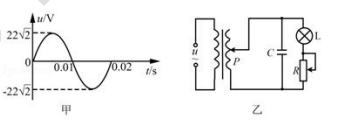$\text{A.}$ 若向下移动 P，电容器的电荷量增 $\text{B.}$ 若保持 P 不动，向下移动 R 的滑片，灯泡变 $\text{C.}$ 若保持 R 的滑片不动，向下移动 P，灯泡变 $\text{D.}$ 电容器的击穿电压为 $11\sqrt{2}V$

D